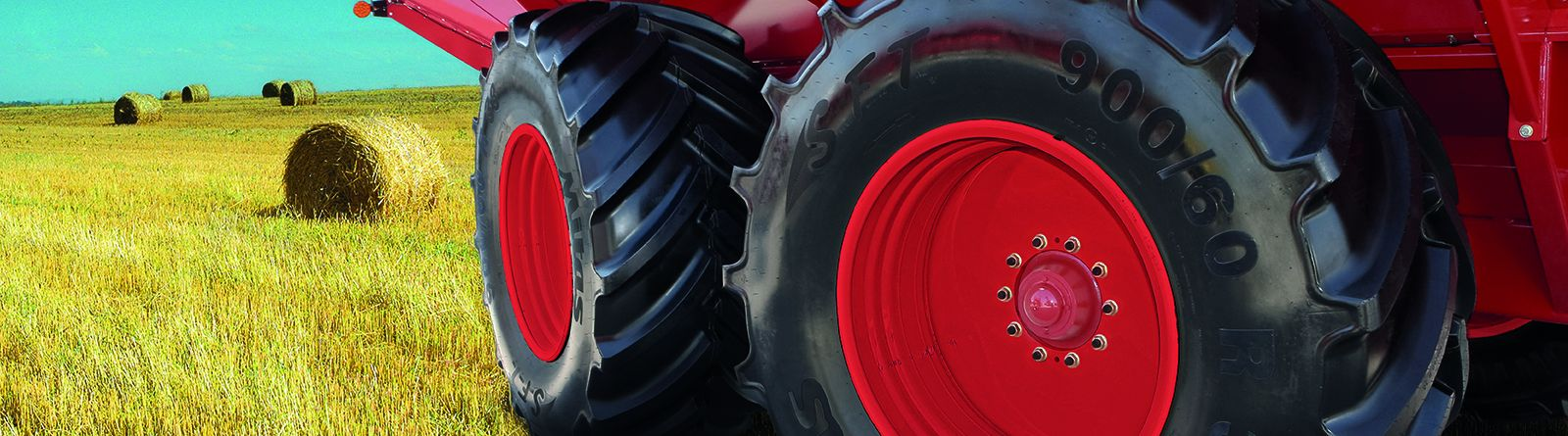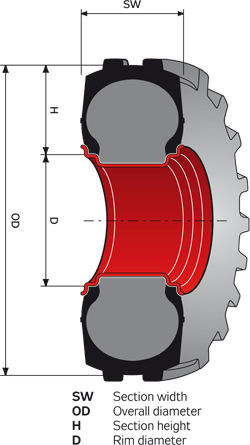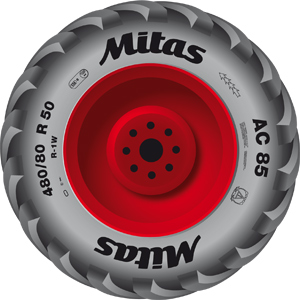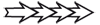# Markings and definitions

## 340/85 R 24

340Nominal section width (in mm)
85Aspect ratio H/SW (in %)
RRadial construction
24Nominal rim diameter (in inches)

## 15.0/55 – 17

15.0Nominal section width (in inches)
55Aspect ratio H/SW (in %)
Cross-ply construction
17Nominal rim diameter (in inches)

## 31 × 15.50 – 15

31Overall diameter (in inches)
15.50Nominal section width (in inches)
Cross-ply construction
15Nominal rim diameter (in inches)

## 17.5 L – 24

17.5Nominal section width (in inches)
LReduced aspect ratio
Cross-ply construction
24Nominal rim diameter (in inches)

## 7.50 – 20

7.50Nominal section width (in inches)
Cross-ply construction
20Nominal rim diameter (in inches)

## 16 × 4

16Overall diameter (in inches)
4Nominal section width (in inches)## Tyre sidewall markingSignMeaning
MITASTrademark of producer
480/65 R 28Tyre size marking
RD-03Tread Pattern code
136Load index (LI 136 = 2 240 kg)
DSpeed symbol (D = 65 km/h)
139Load index (LI 139 = 2 430 kg)
A8Speed symbol (A8 = 40 km/h)
TUBELESSTubeless tyreDirection of rotation
R-1US tread profile marking

Load index (LI 159 = 9 647 lbs)